# Bihar Board 12th Physics Objective Answers Chapter 11 Dual Nature of Radiation and Matter

Bihar Board 12th Physics Objective Questions and Answers

## Bihar Board 12th Physics Objective Answers Chapter 11 Dual Nature of Radiation and Matter

Question 1.
Who established that electric charge is quantised ?
(a) J.J. Thomson
(b) William Crookes
(c) R.A Millikan
(d) Wilhelm Rontgen
(c) R.A MillikanQuestion 2.
Cathode rays were discovered by
(a) Maxwell Clerk James
(b) Heinrich Hertz
(c) William Crookes
(d) J.J. Thomson
(c) William Crookes

Question 3.
The specific charge of a proton is 9.6 × 107C kg-1. The specific charge of an alpha particle will be
(a) 9.6 × 107 C kg-1
(b) 19.2 × 107 C kg-1
(c) 4.8 × 107Ckg-1
(d) 2.4 × 107 C kg-1
(c) 4.8 × 107Ckg-1
Solutin: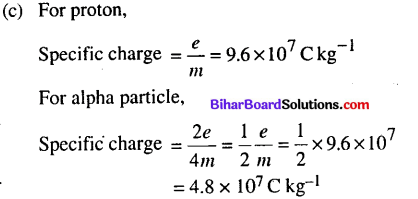Question 4.
Thermionic emissions are related to
(a) conduction
(b) convection
(d) none of these
(b) convectionQuestion 5.
The phenomenon of photoelectric emission was discovered in 1887 by
(a) Albert Einstein
(b) Heinrich Hertz
(c) Wilhelm Hallwaches
(d) Philipp Lenard
(b) Heinrich Hertz

Question 6.
A metal surface ejects electrons when hit by green light but none when hit by yellow light. The electrons will be ejected when the surface is hit by
(a) blue light
(b) heat rays
(c) infrared light
(d) red light
(a) blue light

Question 7.
Which of the following statements is correct regarding the photoelectric experiment ?
(a) The photocurrent increases with intensity of light
(b) Stopping potential increases with increase in intensity of incident light.
(c) The photocurrent increases with increase in frequency.
(d) All of the these.
(a) The photocurrent increases with intensity of light

Question 8.
The maximum value of photoelectric current is called
(a) base current
(b) saturation current
(c) collector current
(d) emitter current
(b) saturation currentQuestion 9.
The time taken by a photoelectron to come out after the photon strikes is approximately
(a) 10-1 s
(b) 10-4 S
(c) 10-10 s
(d) 10-16 s
(c) 10-10 s

Question 10.
Light of wavelengths λ falls on a metal having work function $$\frac{h c}{\lambda_{0}}$$ Photoelectric effect will take place only
(a) λ ≥ λ0
(b) λ ≤ λ0
(c) λ ≥ 2λ0
(d) λ = 4λ0
(b) λ ≤ λ0

Kinetic Energy Calculator calculates the kinetic energy as well as mass and velocity of an object.

Question 11.
The photoelectric cut off voltage in a certain experiment is 1.5 V. The maximum kinetic energy of photoelectron emitted is
(a) 2.4eV
(b) 1.5eV
(c) 3.1eV
(d) 4.5eV
(b) 1.5eV

Question 12.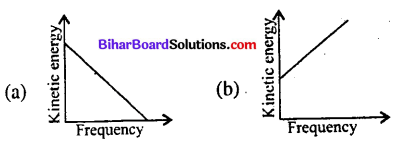(d)Question 13.
Photoelectric emission occurs only when the incident light has more than a certain minimum
(a) power
(b) wavelength
(c) intensity
(d) frequency
(d) frequency

Question 14.
The work function of cesium is 2.14 eV. The threshold frequency of caesium is
(a) 5.16 × 1019 Hz
(b) 5.16 × 1016 Hz
(c) 5.16 × 1018 Hz
(d) 5.16 × 1014 Hz
(d) 5.16 × 1014 Hz
Solution: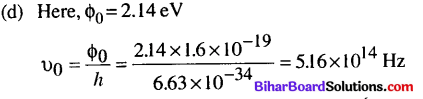Question 15.
Which one among the following shows particle nature of light ?
(a) Photoelectric effect
(b) Interference
(c) Refraction
(d) Polarization
(a) Photoelectric effect

Question 16.
Which of the following statements about photon is incorrect ?
(a) Photons exert no pressure.
(b) Momentum of photon is $$\frac{l v}{c}$$
(c) Rest mass of photon is zero.
(d) Energy of photon is to hυ.
(a) Photons exert no pressure.Question 17.
In a photon-particle collision (such as photon-electron collision), which of the following may not beconserved ?
(a) Total energy
(b) Number of photons
(c) Total momentum
(d) Both (a) and (b)
(b) Number of photons

Question 18.
The rest mass of photon is
(a) $$\frac{h v}{c}$$
(b) $$\frac{h v}{c^{2}}$$
(c) $$\frac{h v}{\lambda}$$
(d) zero
(d) zero

Question 19.
‘n’ photons of wavelength ‘λ’ are absorbed by a black body of mass ‘m ’. The momentum gained by the body is
(a) $$\frac{h}{m \lambda}$$
(b) $$\frac{m n h}{\lambda}$$
(c) $$\frac{n h}{m \lambda}$$
(d) $$\frac{n h}{\lambda}$$
(d) $$\frac{n h}{\lambda}$$

Question 20.
The linear momentum of a 3 MeV photon is
(a) 0.01 eV s m-1
(b) 0.02 eV s m-1
(c) 0.03 eV s m-1
(d) 0.04 eV s m-1
(a) 0.01 eV s m-1
Solution:
(a) Energy of a photon, E = 3 MeV = 3 × 106 eV
Linear momentum of the photon, P = E/c
where c is the speed of light in vacuum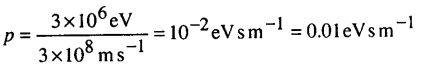Question 21.
The wavelength of matter wave is independent of
(a) mass
(b) velocity
(c) momentum
(d) charge
(d) charge

Question 22.
Which phenomenon best supports the theory that matter has a wave nature ?
(a) Electron momentum
(b) Electron diffraction
(c) Photon momentum
(d) Photon diffraction
(b) Electron diffractionQuestion 23.
The matter-wave picture of electromagnetic wave/ radiation elegantly incorporated the
(a) Heinsenberg’s uncertainty principle
(b) correspondence principle
(c) cosmic theory
(d) Hertz’s observations
(a) Heinsenberg’s uncertainty principle

Question 24.
Wave theory cannot explain the phenomena of
(i) Polarization
(ii) Diffraction
(iii) Compton effect
(iv) Photoelectric effect
Which of the following is correct ?
(a) (i) and (ii)
(b) (ii) and (iv)
(c) (iii) and (iv)
(d) (iv) and (i)
(c) (iii) and (iv)

Question 25.
Which of the following devices is sometimes called an electric eye ?
(a) LED
(b) Photocell
(c) Integrated chip (IC)
(d) Solar cell
(b) Photocell

Question 26.
In which of the following photocell is not used ?
(a) Burglar alarm
(b) Television camera
(c) Automatic street lights
(d) Vacuum cleaner
(d) Vacuum cleanerQuestion 27.
The de Broglie wavelength is given by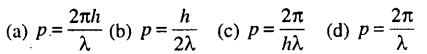(a) $$p=\frac{2 \pi h}{\lambda}$$
Solution:
(a) de Broglie wavelength is given by
$$\lambda=\frac{h}{p}$$, where p is the momentum or $$p=\frac{h}{\lambda}$$
Since, $$\hbar=\frac{h}{2 \pi}$$
.-. $$p=\frac{2 \pi h}{\lambda}$$

Question 28.
Which of these particles having the same kinetic energy has the largest de Broglie wavelength ?
(a) Electron
(b) Alpha particle
(c) Proton
(d) Neutron
(a) Electron

Question 29.
The energy that should be added to an electron to reduce its de Broglie wavelength from one nm to 0.5 nm is
(a) four times the initial energy
(b) equal to the initial energy
(c) twice the initial energy
(d) thrice the initial energy
(d) thrice the initial energy

Question 30.
The de Broglie wavelength associated with a ball of mass 150 g travelling at 30 m s_1 is
(a) 1.47 × 10-34 m
(b) 1.47 × 10-16 m
(c) 1.47 × 10-19 m
(d) 1.47 × 10-31 m
(a) 1.47 × 10-34 m
Solution:
(a) Mass of the ball, m = 150 g = 0.15 kg,
Speed of the ball, v = 30 m s-1
Momentum, p = mv = 0.15 × 30 = 4.5 kg m s-1
de Broglie wavelength,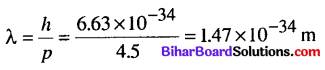Question 31.
A proton and an a-particle are accelerated through the same potential difference. The ratio of de Broglie wavelength λp to that of λα is
(a) √2 : 1
(b) √4 : 1
(c) √6 : 1
(d) √8 :1
(d) √8 :1
Solution:Question 32.
The de Broglie wavelength of a particle of kinetic energy K is λ. What will be the wavelength of the K/4 ?
(a) λ
(b) 2λ
(c) λ/2
(d) 4λ
(b) 2λ

Question 33.
When the velocity of an electron increases, its de Broglie wavelength
(a) increases
(b) decreases
(c) remains same
(d) may increase or decrease
(b) decreasesQuestion 34.
The de Broglie wavelength of a photon is twice the de Broglie wavelength of an electron. The speed of the
electron is ve = c/100 . Then(b) $$\frac{E_{e}}{E_{p}}=10^{-2}$$

Question 35.
The de Broglie wavelength of an electron with kinetic energy 120 eV is (Given h = 6.63 x 10-34 J s, me = 9 x 10-31 kg, 1 eV = 1.6 x 10-19 J)
(a) 2.13 Å
(b) 1.13 Å
(c) 4.15 Å
(d) 3.14 Å
(b) 1.13 Å
Solution:Question 36.
The de Broglie wavelength X of an electron accelerated through a potential V in volts is(a) $$\frac{1.227}{\sqrt{V}} \mathrm{nm}$$
Solution:
(a) Consider an electron of mass m and charge e accelerated from rest through potential V.
Then, K = eV
The de Broglie wavelength X of the electron is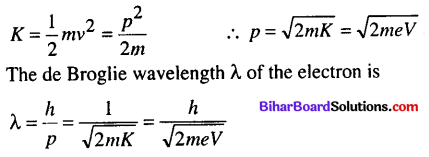Substituting the numerical values of h, m, e, we getQuestion 37.
Electrons with de Broglie wavelength λ fall on the target in an X ray tube. The cut off wavelength (λ0) of the emitted X rays is(a) $$\lambda_{0}=\frac{2 m c \lambda^{2}}{h}$$

Question 38.
G.P. Thomson experimentally confirmed the existence of matter waves by the phenomena
(a) diffraction
(b) refraction
(c) polarization
(d) scattering
(a) diffraction

Question 39.
A particle is dropped from a height H. The de Broglie wavelength of the particle as a function of height is proportional to
(a) H
(b) H1/2
(c) H°
(d) H-1/2
(d) H-1/2
Solution:
(d) Velocity acquired by a particle while falling from a height H is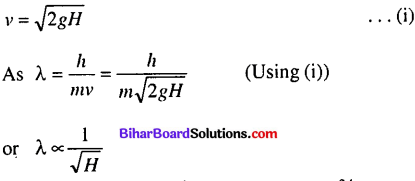Question 40.
The wavelength of a photon needed to remove a proton from a nucleus which is bound to the nucleus with 1 MeV energy is nearly
(a)1.2nm
(b) 1.2 × 10-3 nm
(c) 1.2 × 10-6nm
(d)1.2 × 101nm
(b) 1.2 × 10-3 nm
Solution:Question 41.
A proton, a neutron, an electron and an a-particle have same energy. Then their de Broglie wavelengths compare as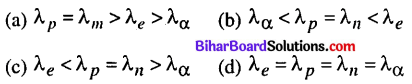(b) $$\lambda_{\alpha}<\lambda_{p}=\lambda_{n}<\lambda_{e}$$
Solution: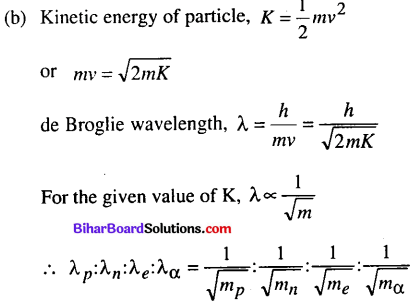Since mp = mn, hence, λp = λn
As ma > mp, therefore λα < λp
As me < mn, therefore λe > λn
Hence λα < λp=λn < λeQuestion 42.
An electron is moving with an initial velocity $$\vec{v}=v_{0} \hat{i}$$ and is in a magnetic field $$\vec{B}=B_{0} \hat{j}$$ Then it’s de Broglie wavelength
(a) remains constant
(b) increases with time
(c) decreases with time
(d) increases and decreases periodically